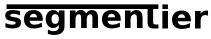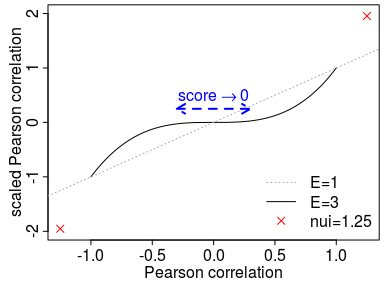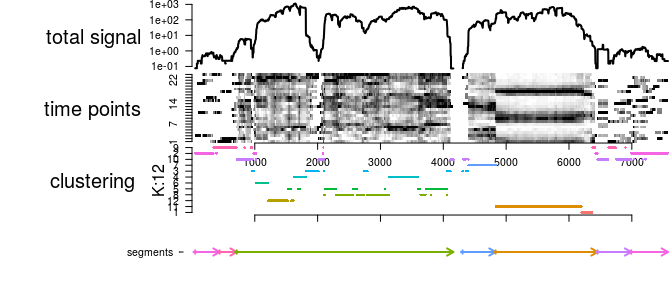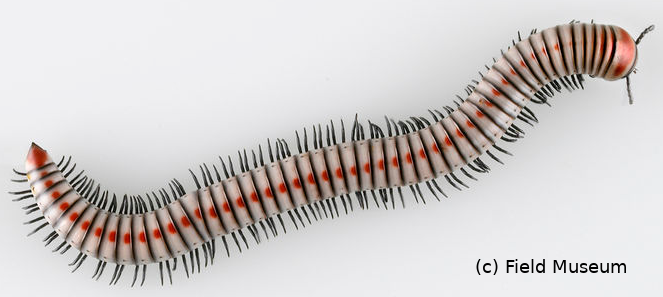# SummarysegmenTier is a dynamic programming solution to segmentation based on maximization of arbitrary similarity measures within segments as developed in Machné, Murray, and Stadler (2017).

In addition to the core algorithm, function segmentClusters, the package provides time-series processing and clustering functions as described in the publication. These are generally applicable where a k-means clustering yields meaningful results, and have been specifically developed for clustering of the Discrete Fourier Transform of periodic gene expression data (“circadian” or “yeast metabolic oscillations”).

This clustering approach is outlined in the supplemental material of Machné and Murray (2012), and here is used as a basis of segment similarity measures. Note, that the functions processTimeseries and clusterTimeseries, can also be used as stand-alone tools for periodic time-series analysis and clustering.

# Theory & Implementation

The ideas and theory behind the package are detailed in Machné, Murray, and Stadler (2017), here we provide a synopsis.

## The Recursion

segmenTier’s input is a clustering $$\mathcal{C}_{\alpha}\subseteq\mathbb{X}$$, $$\alpha=1,\dots n$$. segmenTier then solves the recursion:

\begin{equation} S_{k,\alpha} = \max_{j\le k} \max_{\beta\ne\alpha} S_{j-1,\beta} + s(j,k,\alpha) - M\;, \label{eq:01} \end{equation}

where $$s(j,k,\alpha)$$ is a scoring function that measures the similarity of a segment, from positions $$j$$ to $$k$$, to cluster $$\mathcal{C}_\alpha$$, and $$M$$ is a penalty incurred by the use of a new segment, a fixed cost for each jump that allows to fine-tune minimal segment lengths. The algorithm maximizes scores for breakpoints at which maximal inter-segment similarities are reached when switching between two distinct clusters at positions $$j$$ (“$$\max_{\beta\ne\alpha}$$”). This recursion is implemented in Rcpp for efficiency, and directly available as function calculateScore.

Back-tracing the maximal scores $$S_{k,\alpha}$$, function backtrace, then provides both segment borders and segment cluster associations.

The main interface function segmentClusters wraps the recursion and back-tracing functions and handles scoring function selection and additional parameters.

## The Scoring Functions

Three scoring functions are available. They all sum up a similarity measure between positions $$j$$ and $$k$$ and clusters $$\mathcal{C}$$.

The first two rely on a clustering of all positions $$x$$

\begin{equation} s(j,k,\alpha) = \sum_{i=j}^k Q(\mathcal{C}_i,\mathcal{C}_\alpha) \end{equation}

where $$\mathcal{C}_i$$ is the cluster label of data row $$x_i$$, and $$Q(\mathcal{C},\mathcal{D})$$ is an, in principle arbitrary, similarity measure for the two clusters. The most basic choice is $$Q(\mathcal{C},\mathcal{C})=1$$ and $$Q(\mathcal{C},\mathcal{D})=a<0$$ for $$\mathcal{C}\ne\mathcal{D}$$. This case is available as scoring function “ccls” (argument S="ccls") with a default value $$a=-2$$, and requires as sole input a vector of cluster labels.

For our application, an RNA-seq time-series, the Pearson correlation between the cluster centroids (mean values) proofed useful: $$Q(\mathcal{C},\mathcal{D})= \text{corr}(\bar x_{\mathcal{C}},\bar x_{\mathcal{D}})$$ is pre-calculated as a cluster-cluster correlation matrix by segmenTier’s interface to kmeans clustering (clusterTimeseries), and available as scoring function “ccor” (S="ccor").

The third option does not rely on a clustering of all positions and allows to use cluster centroids that are independently derived, eg. from only a subset of the data. The scoring function

\begin{equation} s(j,k,\alpha) = \sum_{i=j}^k \tilde\sigma(x_i,\mathcal{C}_{\alpha}) \end{equation}

measures the similarity of each data row $$x_i$$ to a cluster centroid, is again implemented as Pearson correlations, $$\tilde\sigma(x_i,\mathcal{C}_{\alpha})= \text{corr}(x_i,\bar x_{\mathcal{C}_{\alpha}})$$, pre-calculated by the clustering interface, and available as scoring function “icor” (S="icor").

For efficiency, Pearson correlations are calculated in a custom function in Rcpp. Note that it is not necessary to pre-calculate all values of $$s(i,k,\mathcal{C}$$, since we can simply subtract sums; for $$i>1$$:

\begin{equation} s(j,k,\alpha)= s(1,k,\alpha) - s(j-1,k,\alpha)\;. \end{equation}

In summary, the primitive scoring function “ccls” requires only a vector of cluster labels as input. Scoring function “ccor” requires both a vector of cluster labels and a cluster-cluster similarity matrix, and scoring function “icor” requires only a position-cluster similarity matrix. These matrices, internally called csim, are provided by segmenTier’s clustering function clusterTimeseries using Pearson correlations to cluster centroids, but can also be provided by the user, as outlined in section User-Defined Similarities.

## Scaling & Nuisance Segments

It proofed useful to further emphasize correlation-based similarities by an exponent $$\epsilon>1$$, which further weakens moderate positive or negative correlations, and this is available as argument E for all scoring functions. Signs are preserved, allowing for even-valued exponents.

Additionally, one can define a nuisance cluster in pre-processing of the data, eg. total data values or data-cluster correlations below a certain threshold, and enforce such segments by using a higher similarity in combination with a lower length penalty (argument Mn). Argument nui of segmentClusters will be the self-similarity of the nuisance segment, and -nui the nuisance similarity to all other clusters. The exponent E will also be applied to nuisance similarity nui.

The figure below shows the effects of E>1 on Pearson correlations and nui>1, ie. on the socring function. Detailed analysis of the effects of these parameters and the length penalty M on segmentation is provided in Machné, Murray, and Stadler (2017) and demonstrated in the R demo “segment_data”.## User-Defined Similarities

segmenTier’s clustering interface (clusterTimeseries) pre-calculates the cluster-cluster (“ccor”) and position-cluster (“icor”) similarity matrices and adds them to the “clustering” object, list item csim in the object that is passed to the recursion function as a look-up table.

Advanced users can provide similarity measures themselves by constructing csim where an input cluster labeling (argument seq) must be integers that serve as column and row indices of this matrix, ie. similarity between a cluster “2” and a cluster “3” is stored in csim[2,3] for scoring function “ccor”.

For scoring function “icor” columns are also indexed by cluster labels, and rows are the indices at positions $$i$$, ie. csim[345,4] is the similarity of data row 345 to the cluster labeled “4”. Scoring function “icor” in principle does not require cluster labels for all positions. However, in the current implementation a cluster label vector must still be supplied. It can consist of arbitrary values, EXCEPT for positions pre-determined as “nuisance” with fixed similarities nui. At such position the cluster label should be “0”.

Scaling E and nuisance similarities nui can be applied to user-defined similarity matrices, but when choosing E=1 (default) and simply NOT supplying any clusters labels “0” they will have no effect.

In summary, users that wish to define their own similarities are best advised to provide these similarities as a numeric matrix, argument csim in the main function segmentClusters. The reported cluster labels of segments are the column indices of csim. For scoring function “ccor” each position must be assigned to a cluster label and csim is a cluster-cluster similarity matrix. For scoring function “icor”, recommended for this custom use of the algorithm, csim is a position-cluster similarity matrix and cluster labels in argument seq allow to define a nuisance cluster. Construction of csim is demonstrated in the R demo segment_test.

## Time-Series Processing & Clustering

Segmentation by custom similarities is simple, see section User-Defined Similarities. However, segmenTier provides a pipeline of time-series processing and clustering functions that is to some extent specific to the data for which the algorithm was developed, but should also be generally applicable.

The function processTimeseries prepares a time-series, with time points in columns, and individual measurements in rows, for segmentation. The function produces a list, an S3 object of class “timeseries”, that serves as input for the clustering function. It is generally applicable to provide the input for the clustering wrapper, and raw input data will be clustered and segmented when setting options use.fft=FALSE and trafo="raw", and use na2zero=TRUE to avoid interpretation of NA values as 0 (which is useful for positive valued signals where absence of data means absence of signal, eg. sequencing-based data (“read-counts”)).

The algorithm had been originally designed for periodic data, and processTimeseries can also perform a Discrete Fourier Transform (DFT, use.fft=TRUE) using the stats package function mvfft, including a permutation analysis (perm>0) that provides p-values for all periodic components for the DFT. Clustering of the DFT of periodic data works well to distinguish time-courses with similar temporal profiles. This approach has been applied to microarray-based transcriptome data from “metabolic” or “respiratory oscillations” in budding yeast (Machné and Murray 2012) and “diurnal” or “circadian oscillations” of cyanobacteria (Lehmann et al. 2013, Beck et al. (2014)). This approach is implemented in the function clusterTimeseries, using a simple k-means clustering of the passed “timeseries” object.

In the original DFT-based clustering approach a better clustering of the DFT of periodic data was obtained by using a model-based clustering algorithm that allows for tailed distributions, as implemented in the BioConductor package flowClust. This is available in the function flowclusterTimeseries. However, this is much slower than k-means and not recommended in the context of segmentation. Future implementations will fuse different clustering methods in one function, but likely be implemented in a distinct R package (see clusterTimeseries2 in github package segmenTools).

However, within segmenTier the output of clusterTimeseries, S3 object of class “clustering”, serves as input for segmentation and provides the similarity matrices required for scoring functions “icor” and “ccor”. The correct matrix is automatically selected by segmentClusters.

## Package Outlook

The next development cycle of this package should allow for more efficient sweeping of “long” data sets, eg. genome-wide data:

• More generic function to provide input for segmentation, eg.
• A function that takes cluster centers and data as inputs and provides a “clustering” object with csim similarity matrices for segmentClusters, and
• A maximal length parameter in the recursion, to sweep across long data-sets, removing the pre-segmentation requirement,
• A subset clustering utility, that samples a reasonable subset of the data for clustering, and supplies cluster centers.

# Usage

## Installation

From CRAN:

install.packages("segmenTier")

The development version can be obtained from github using devtools:

library(devtools)
install_github("raim/segmenTier", subdir = "pkg")

## Quick Guide

library(segmenTier)

data(primseg436) # RNA-seq time-series data

# Fourier-transform and cluster time-series:
tset <- processTimeseries(ts=tsd, na2zero=TRUE, use.fft=TRUE,
dft.range=1:7, dc.trafo="ash", use.snr=TRUE)
cset <- clusterTimeseries(tset, K=12)
## Timeseries N 7624
## Used datapoints  7374
## Clusters K   12
# ... segment it:
segments <- segmentClusters(seq=cset, M=100, E=2, nui=3, S="icor")
## Scoring matrix   20190209 14:04:17
## parameters   function:icor; scale:2; max/min:max
## Backtracing  20190209 14:04:24
## parameters   multib:max
## elapsed, sec 6
## Done at      20190209 14:04:24
# and inspect results:
plotSegmentation(tset, cset, segments, cex=.5, lwd=2)print(segments)
##
## Similarity-based segmentation by dynamic programming:
## Total length:  7624
## Segments:
##      CL start  end
## [1,]  2    52  439
## [2,]  9   440  712
## [3,]  8   713 4160
## [4,]  7  4306 4831
## [5,] 11  4832 6455
## [6,] 10  6456 6989
## [7,]  2  6990 7574
##
## Parameters:
##          k    S E   M Mn  a nui multi nextmax multib
## segments 1 icor 2 100 20 -2   3   max    TRUE    max
##
## Run time:  6.226 seconds
## and get segment border table for further processing
head(segments\$segments)
##      CL start  end
## [1,]  2    52  439
## [2,]  9   440  712
## [3,]  8   713 4160
## [4,]  7  4306 4831
## [5,] 11  4832 6455
## [6,] 10  6456 6989

# Demonstrations

Usage of the package is further demonstrated in two R demos.

## Demo I: Direct Interface to Algorithm

The main low level interface to the algorithm, function segmentClusters, is demonstrated in the file demo/segment_test.R. It produces Supplemental Figure S1 of Machné, Murray, and Stadler (2017).

To run it as a demo in R simply type:

demo("segment_test", package = "segmenTier")

## Demo II: Clustering, Batch Segmentation & Parameter Scans

A real-life data set is processed, clustered and segmented with varying parameters in demo/segment_data.R.

This demo runs quite long, since it calculates many segmentations. It provides a comprehensive overview of the effects of segmentation parameters E, M and nui, and produces (among others) Figure 3 and Supplemental Figures S4a and S4b of Machné, Murray, and Stadler (2017).

demo("segment_data", package = "segmenTier")

# Karl, the segmenTier